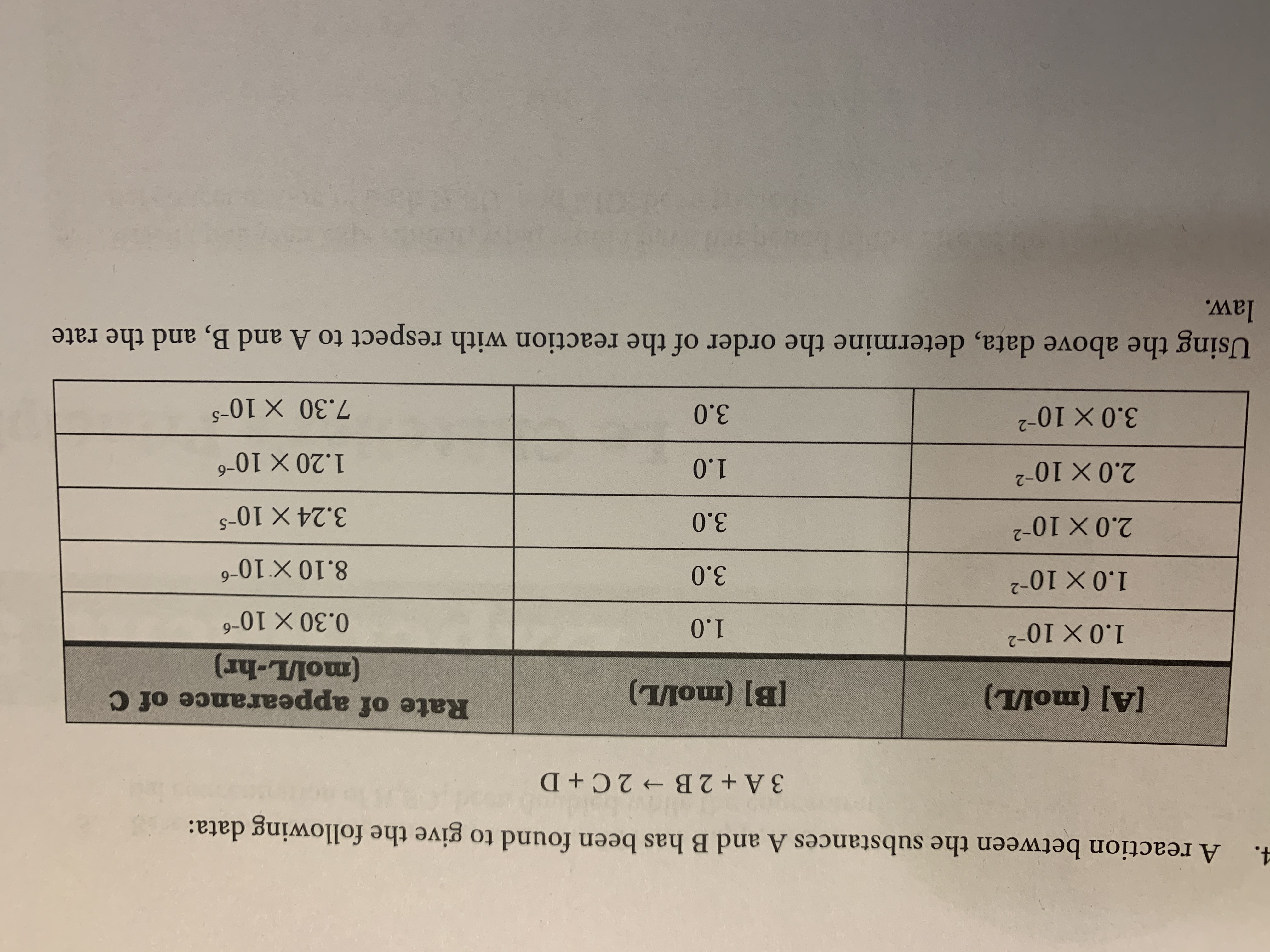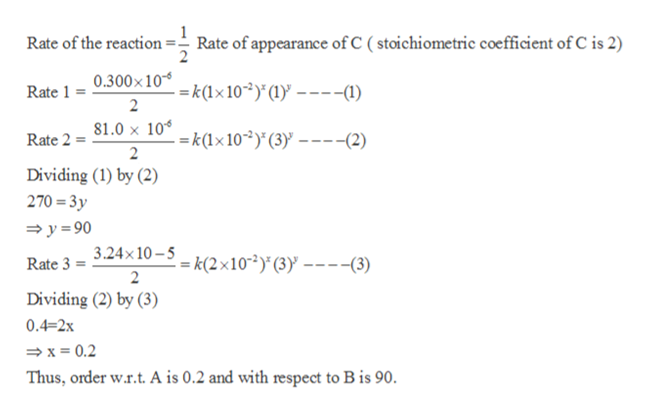# 4.A reaction between the substances A and B has been found to give the following data:3 A+2 B 2 C + DRate of appearance of C(тлош) [v](тлош) [al1.0 X 10-21.00.30 X 10-61.0 X 103.08.10 X 10-62.0 x 1023.03.24 X 10-2.0 x 1021.20 x 1069-0'I3.0 X 1027.30 X 105Using the above data, determine the order of the reaction with respect to A and B, and the ratelaw.

Question
1 viewshelp_outlineImage Transcriptionclose4. A reaction between the substances A and B has been found to give the following data: 3 A+2 B 2 C + D Rate of appearance of C (тлош) [v] (тлош) [al 1.0 X 10 -2 1.0 0.30 X 10-6 1.0 X 10 3.0 8.10 X 10-6 2.0 x 102 3.0 3.24 X 10- 2.0 x 102 1.20 x 106 9- 0'I 3.0 X 10 2 7.30 X 105 Using the above data, determine the order of the reaction with respect to A and B, and the rate law. fullscreen
check_circle

Step 1

a. Let the rate law for the reaction be :

Rate = k [A] x [B] y

where,

k = rate constant for the reaction

x = order with respect to A

y = order with respect to Bhelp_outlineImage TranscriptioncloseRate of the reaction- Rate of appearance of C ( stoichiometric coefficient of C is 2) Rate 1 0.300x10* =k(1x10~2)°(1)y ----1) 2 Rate 2=81.0 x 10° =k(1x10~2)°(3) -(2) 2 Dividing (1) by (2) 270 3y y90 Rate 3 = 3.24x 10-3 = k(2x10^2)*(3) ----(3) 2 Dividing (2) by (3) 0.4-2x x 0.2 Thus, order w.r.t. A is 0.2 and with respect to B is 90. fullscreen
Step 2

b) Rate law :

Rate = k [A]0.2 ...

### Want to see the full answer?

See Solution

#### Want to see this answer and more?

Solutions are written by subject experts who are available 24/7. Questions are typically answered within 1 hour.*

See Solution
*Response times may vary by subject and question.
Tagged in

### General Chemistry